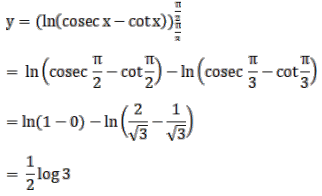# Mark against the correct answer in the following:

Question:

Mark $(\sqrt{)})$ against the correct answer in the following:

$\int_{\pi / 3}^{\pi / 2} \operatorname{cosec} x d x=?$

A. $\frac{1}{2} \log 2$

B. $\frac{1}{2} \log 3$

C. - $\log 2$

D. none of these

Solution: